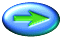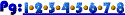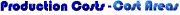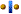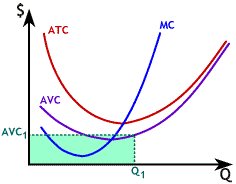We will find it very useful to be able to show Total Cost (TC) and Total Variable Cost (TVC) as areas on our graphs of cost curves.

We computed AVC as TVC ÷ Q. At any level of output, the height of the AVC curve is Average Variable Cost for that level of output. This means that, for any given level of output, TVC = AVC x Q

At Q1the height of the AVC curve, AVC1, gives Average Variable Cost for Q1 units of output. So, TVC at Q1 is just TVC1 = AVC1 x Q1 and is shown as the light green shaded area. The tan shaded area shows TVC at Q2, a higher level of output.